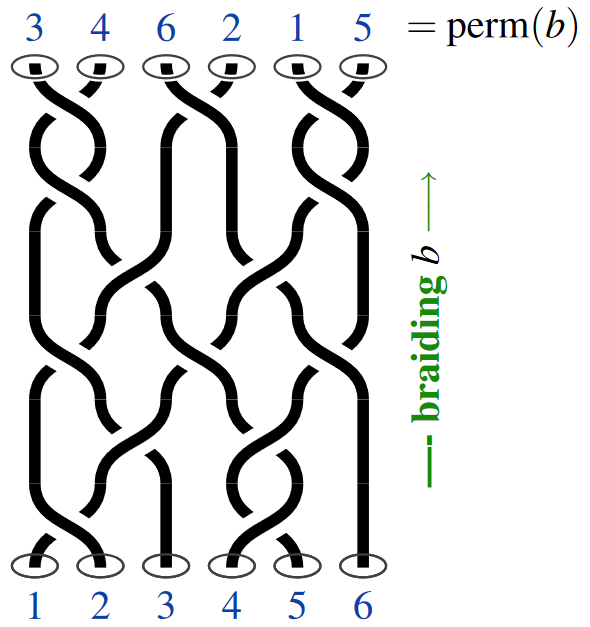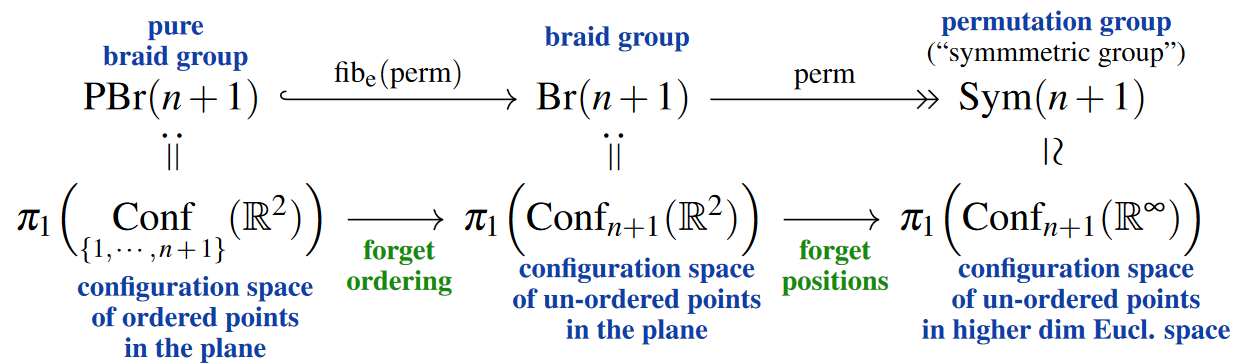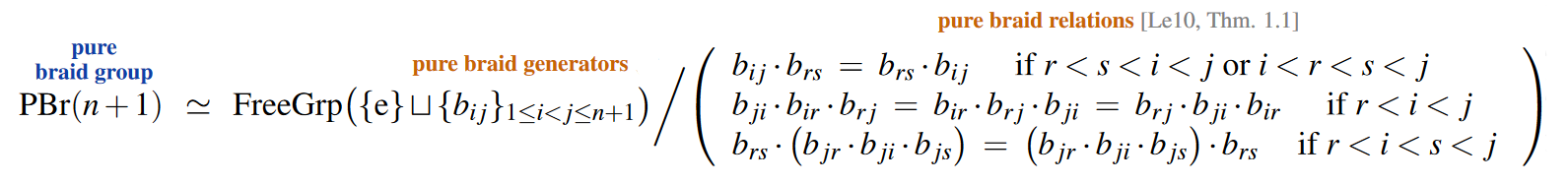Contents

group theory

# Contents

## Idea

By a braid group [Artin (1925)] one means the group of joint continuous motions [cf. Goldsmith (1981)] of a fixed number $n+1$ of non-coincident points in the plane, from any fixed configuration back to that fixed configuration. The “worldlines” traced out by such points in space-time under such an operation look like a braid with $n+1$ strands, whence the name.As with actual braids, here it is understood that two such operations are identified if they differ only by continuous deformations of the “strands” without breaking or intersecting these, hence that one identifies those such systems of worldlines which are isotopic in $\mathbb{R}^3$. (This is just the kind of invariance considered for link diagrams — such as under Reidemeister moves — and in fact every link diagram may be obtained by “closing up” a braid diagram, in the evident sense.)

A quick way of saying this with precision is to observe that a braid group is thus the fundamental group $\pi_1$ of a configuration space of points in the plane (see below for more).

Here it makes a key difference whether:

• one considers the points in a configuration as ordered (labeled by numbers $1, \cdots, n+1$) in which case one speaks of the pure braid group,

• or as indistinguishable (albeit in any case with distinct positions!) in which case one speaks of the braid group proper.

Namely, after traveling along a general braid $b$ the order of the given points may come out permuted by a permutation $\mathrm{perm}(b)$, and the braid is called pure precisely if this permutation is trivial:More explicitly, braid groups admit finite presentations by generators and relations. To these we now turn first:

## Definitions and characterizations

#### Via generators and relations

##### Presentation of general braids

We discuss the braid group as a finitely generated group (Artin 1925, (5)-(6); Artin 1947, (18)-(19); review in, e.g.: Fox & Neuwirth 1962, §7):

###### Definition

(Artin presentation)

The Artin braid group, $Br({n+1})$, on $n+1$ strands is the finitely generated group given via generators and relations by (this and the following graphics are taken from Myers et al. (2023)):

• generators:

(1)$b_i\;\; i = 1, \ldots, n$
• relations I:

(2)$\underset{ i+1 \lt j }{\forall} \;\;\; b_i \cdot b_j \;=\; b_j \cdot b_i$

• relations II

$\underset{ 1 \leq i \lt n }{\forall} \;\;\; b_i \cdot b_{i+1} \cdot b_i \;=\; b_{i+1} \cdot b_i \cdot b_{i+1}$

##### Presentation of pure braids

Similarly, the pure braid group has a finite presentation.

One possible set of generators (also originally considered by Artin) are the “weave” braids where one strands lassos exactly one other strand:

In terms of these generators, the pure braid group is obained by quotienting out the following relations — an optimization of Artin’s original pure braid relations, due to Lee (2010, Thm. 1.1, Rem. 3.1):Here we repeatedly used products of pure braid generators of the following form:

#### As fundamental group of a configuration space of points

Geometrically, one may understand the group of braids in $\mathbb{R}^3$ as the fundamental group of the configuration space of points in the plane $\mathbb{R}^2$ (traditionally regarded as the complex plane $\mathbb{C}$ in this context, though the complex structure plays no role in the definition of the braid group as such).

(originally due to Hurwitz 1891, §II, then re-discovered/re-vived in Fadell & Neuwirth 1962, p. 118, Fox & Neuwirth 1962, §7, review includes Birman 1975, §1, Williams 2020, pp. 9)

In the plane

We say this in more detail:

Let $C_n \hookrightarrow \mathbb{C}^n$ denote the space of configurations of n ordered points in the complex plane, whose elements are those n-tuples $(z_1, \ldots, z_n)$ such that $z_i \neq z_j$ whenever $i \neq j$. In other words, $C_n$ is the complement of the fat diagonal:

$C_n \;\coloneqq\; \mathbb{C}^n \setminus \mathbf{\Delta}^n_{\mathbb{C}} \,.$

The symmetric group $S_n$ acts on $C_n$ by permuting coordinates. Let:

• $C_n/S_n$ denote the quotient by this group action, hence the orbit space (the space of $n$-element subsets of $\mathbb{C}$ if one likes),

• $[z_1, \ldots, z_n]$ denote the image of $(z_1, \ldots, z_n)$ under the quotient coprojection $\pi \colon C_n \to C_n/S_n$ (i.e. its the equivalence class).

We understand $p = (1, 2, \ldots, n)$ as the basepoint for $C_n$, and $[p] = [1, 2, \ldots n]$ as the basepoint for the configuration space of unordered points $C_n/S_n$, making it a pointed topological space.

###### Definition

The braid group is the fundamental group of the configuration space of n unordered points:

$Br(n) \;\coloneqq\; \pi_1 \big( C_n/S_n, [p] \big)$

The pure braid group is the fundamental group of the configuration space of n ordered points:

$PBr(n) \;\coloneqq\; \pi_1 \big( C_n, p \big) \,.$

Evidently a braid $\beta$ is represented by a path $\alpha: I \to C_n/S_n$ with $\alpha(0) = [p] = \alpha(1)$. Such a path may be uniquely lifted through the covering projection $\pi: C_n \to C_n/S_n$ to a path $\tilde{\alpha}$ such that $\tilde{\alpha}(0) = p$. The end of the path $\tilde{\alpha}(1)$ has the same underlying subset as $p$ but with coordinates permuted: $\tilde{\alpha}(1) = (\sigma(1), \sigma(2), \ldots, \sigma(n))$. Thus the braid $\beta$ is exhibited by $n$ non-intersecting strands, each one connecting an $i$ to $\sigma(i)$, and we have a map $\beta \mapsto \sigma$ appearing as the quotient map of an exact sequence

$1 \to PBr(n) \to Br(n) \to Sym(n) \to 1$

which is part of a long exact homotopy sequence corresponding to the fibration $\pi \colon C_n \to C_n/S_n$.

In general surfaces or graphs

Since the notion of a configuration space of points makes sense for points in any topological space, not necessarily the plane $\mathbb{R}^2$, the above geometric definition has an immediate generalization:

For $\Sigma$ any surface, the fundamental group of the (ordered) configuration space of points in $\Sigma$ may be regarded as generalized (pure) braid group. These surface braid groups are of interest in 3d topological field theory and in particular in topological quantum computation where it models non-abelian anyons.

Yet more generally, one may consider the fundamental group of the configuration space of points of any topological space $X$.

For example for $X$ a 1-dimensional CW-complex, hence an (undirected) graph, one speaks of graph braid groups (e.g. Farley & Sabalka 2009).

The following should maybe not be here in the Definition-section, but in some Properties- or Examples-section, or maybe in a dedicated entry on graph braid groups?:

It has been shown (An & Maciazek 2006, using discrete Morse theory and combinatorial analysis of small graphs) that graph braid groups are generated by particular particle moves with the following description:

1. Star-type generators: exchanges of particle pairs on vertices of the particular graph

2. loop type generators: circular moves of a single particle around a simple cycle of the graph

#### As mapping class group of punctured disk

The braid group $Br(n)$ may be alternatively described as the mapping class group of a 2-disk $D^2$ with $n$ punctures.

Concretely, consider

1. $D^2 \setminus \{z_1, \cdots, z_n\}$

denoting the complement of $n$ distinct points in the closed disk (with boundary the circle);

2. $Homeo^{\partial}\big(D^2 \setminus \{z_1, \cdots, z_n\} \big)$

denoting the mapping space of auto-homeomorphisms which restrict to the identity on the boundary circle, regarding with its canonical group structure;

3. $Homeo^{\partial}_id\big(D^2 \setminus \{z_1, \cdots, z_n\} \big)$

denoting the subgroup which is the connected component of the identity.

Then the mapping class group is

$MCG \big( D^2 \setminus \{z_1, \cdots, z_n\} \big) \;\coloneqq\; Homeo^{\partial}\big(D^2 \setminus \{z_1, \cdots, z_n\} \big) \Big/ Homeo^{\partial}_{id}\big(D^2 \setminus \{z_1, \cdots, z_n\} \big) \,.$

Now observe that

1. for the case that $n = 0$ this group is trivial, by Alexander's trick.

2. continuous extension yields an injection

$Homeo^{\partial}\big(D^2 \setminus \{z_1, \cdots, z_n\} \big) \xhookrightarrow{\phantom{-}\iota\phantom{-}} Homeo^{\partial}\big(D^2\big) \,.$

Combining this implies that for every $[\phi] \,\in\, MCG\big(D^2 \setminus \{z_1, \cdots, z_n\}big)$ therese is an isotopy of $\iota[\phi] \to id$ under which the locations of the punctures trace out a braid (in the sense of a loop in the symmetrized configuration space of points). This construction yields a morphism from the mappign class group? to the braid group

$MCG \big( D^2 \setminus \{z_1, \cdots, z_n\} \big) \xrightarrow{\;\;\sim\;\;} Br(n) \,.$

and this is an isomorphism.

###### Remark

(action of braid group on fundamental group of $n$-punctured disk)
It follows in particular that the braid group $Br(n)$ has a canonical group action on the fundamental group of the $n$-punctured disk. Since the latter is isomorphic to the free group on $n$ generators, this leads to the purely algebraic characterization of the braid group below.

#### As automorphisms of a free group

Since the fundamental group of $D^2 \setminus \{z_1, \cdots, z_n\}$ is the free group of $n$ generators, the MCG-presentation of the braid group (above) induces a group homomorphism of the braid group into the automorphism group of a free group, which turns out to be faithful.

This presentation is due to Artin 1925, §6, review includes González-Meneses 2011, §1.6, see also pointer in Bardakov 2005, p. 2.

More in detail, since the homotopy type of this punctured disk is, evidently, that of the the wedge sum of $n$ circles, it follows that its fundamental group is is the free group $F_n$ on $n$ generators:

$\pi_1 \big( D^2 \setminus \{z_1, \cdots, z_ n\} \big) \;\simeq\; \pi_1 \big( \vee_n S^1 \big) \;\simeq\; F_n \,.$

Now the functoriality of $\pi_1 \,\colon\, Top^{\ast/} \longrightarrow Grp$ implies we have an induced homomorphism

$Aut \big( D^2 \setminus \{z_1, \cdots, z_n\} \big) \longrightarrow Aut \Big( \pi_1\big( D^2 \setminus \{z_1, \cdots, z_n\} \big) \Big) \,\simeq\, Aut(F_n) \,.$

If such an automorphism $\phi$ of $D^2 \setminus \{z_1, \cdots, z_n\}$ is isotopic to the identity, then of course $\pi_1(\phi)$ is trivial, which means that the above homomorphism factors through the quotient group known as the mapping class group:

$MCG \big( D^2 \setminus \{z_1, \cdots, z_n\} \big) \,=\, Aut \big( D^2 \setminus \{z_1, \cdots, z_n\} \big)/Aut_0 \big( D^2 \setminus \{z_1, \cdots, z_n\} \big)$

Therefore, the above gives a homomorphism of the following form, which turns out to be a monomorphism:

$Br(n) \,=\, MCG \big( D^2 \setminus \{z_1, \cdots, z_n\} \big) \xhookrightarrow{\phantom{--}} Aut(F_n)$

Explicitly, the generator $yb_i$ (1) in the Artin presentation(Def. ) is mapped to the automorphism $\sigma_i$ on the free group on $n$ generators $t_1, \ldots, t_n$ which is given as follows:

$\sigma_i(t_j) \,=\, \left\{ \begin{array}{lcl} t_{i+1} & \vert & j = i \\ t_{i+1}^{-1} \cdot t_i \cdot t_{i+1} & \vert & j = i + 1 \\ t_j &\vert& \text{otherwise.} \end{array} \right.$

## Properties

### Relation to moduli space of monopoles

###### Proposition

(moduli space of monopoles is stably weak homotopy equivalent to classifying space of braid group)

For $k \in \mathbb{N}$ there is a stable weak homotopy equivalence between the moduli space of k monopoles (?) and the classifying space of the braid group $Br({2k})$ on $2 k$ strands:

$\Sigma^\infty \mathcal{M}_k \;\simeq\; \Sigma^\infty Br({2k})$

## Examples

The first few examples of the braid group $Br(n)$ for low values of $n$:

###### Example

The group $Br(1)$ has no generators and no relations, so is the trivial group:

$Br(1) \;\simeq\; 1 \,.$

###### Example

The group $Br(2)$ has one generator and no relations, so is the infinite cyclic group of integers:

$Br(2) \;\simeq\; \mathbb{Z} \,.$

###### Example

The group $Br(3)$ (we will simplify notation writing $u = y_1$, $v = y_2$) has presentation

$Br(3) \;\simeq\; \mathcal{P} \coloneqq \big( u,v : r \equiv u v u v^{-1} u^{-1} v^{-1} \big).$

This is also known as the “trefoil knot group”, i.e., the fundamental group of the complement of a trefoil knot.

###### Example

The group $Br(4)$ (simplifying notation as before) has generators $u,v,w$ and relations:

• $r_u \equiv v w v w^{-1} v^{-1} w^{-1}$,

• $r_v \equiv u w u^{-1} w^{-1}$,

• $r_w \equiv u v u v^{-1} u^{-1} v^{-1}$.

###### Example

The Hurwitz braid group (or sphere braid group) is the surface braid group for $\Sigma$ the 2-sphere $S^2$. Algebraically, the Hurwitz braid group $H_{n+1}$ has all of the generators and relations of the Artin braid group $Br({n+1})$, plus one additional relation:

$y_1 y_2 \dots y_{n-1} y_n^2 y_{n-1}\dots y_2 y_1$

### General

The braid group regarded as the fundamental group of a configuration space of points is considered (neither of them under these names, though) already in:

there regarded as acting on Riemann surfaces forming branched covers, by movement of the branch points.

The original articles dedicated to analysis of the braid group:

Survey of the early history:

The understanding of the braid group as the fundamental group of a configuration space of points was re-discovered/re-vived (after Hurwitz 1891) in:

Textbook accounts:

Further introduction and review:

As an example of motion general “motion groups” (such as loop braid groups):

Algebraic presentation of braid groups:

More finite presentations of the pure braid group:

More on the relation of braid groups to mapping class groups:

More on the braid representation on automorphisms of free groups:

On the group homology and group cohomology of braid groups:

Relation of automorphism groups of the profinite completion of braid groups to the Grothendieck-Teichmüller group:

On orderings of the braid group:

• Patrick Dehornoy, Braid groups and left distributive operations , Transactions AMS 345 no.1 (1994) pp.115–150.

• H. Langmaack, Verbandstheoretische Einbettung von Klassen unwesentlich verschiedener Ableitungen in die Zopfgruppe , Computing 7 no.3-4 (1971) pp.293-310.

On geometric presentations of braid groups:

• Byung Hee An, Tomasz Maciazek, Geometric Presentations of Braid Groups for Particles on a Graph, Communications in Mathematical Physics 384 (2021) 1109-1140 [doi:10.1007/s00220-021-04095-x]

On G-structure for $G = Br_\infty$ the infinite braid group:

### Braid group representations (as topological quantum gates)

On linear representations of braid groups (see also at braid group statistics and interpretation as quantum gates in topological quantum computation):

Review:

in relation to modular tensor categories:

• Colleen Delaney, Lecture notes on modular tensor categories and braid group representations, 2019 (pdf, pdf)

Braid representations from the monodromy of the Knizhnik-Zamolodchikov connection on bundles of conformal blocks over configuration spaces of points:

and understood in terms of anyon statistics:

Braid representations seen inside the topological K-theory of the braid group‘s classifying space:

• R. B. Zhang, Braid group representations arising from quantum supergroups with arbitrary $q$ and link polynomials, Journal of Mathematical Physics 33, 3918 (1992) (doi:10.1063/1.529840)

Introduction and review:

Realization of Fibonacci anyons on quasicrystal-states:

• Marcelo Amaral, David Chester, Fang Fang, Klee Irwin, Exploiting Anyonic Behavior of Quasicrystals for Topological Quantum Computing, Symmetry 14 9 (2022) 1780 $[$arXiv:2207.08928, doi:10.3390/sym14091780$]$

Realization on supersymmetric spin chains:

• Indrajit Jana, Filippo Montorsi, Pramod Padmanabhan, Diego Trancanelli, Topological Quantum Computation on Supersymmetric Spin Chains $[$arXiv:2209.03822$]$

### Graph braid groups

• Daniel Farley, Lucas Sabalka, Presentations of Graph Braid Groups (arXiv:0907.2730)

• Ki Hyoung Ko, Hyo Won Park, Characteristics of graph braid groups (arXiv:1101.2648)

• Byung Hee An, Tomasz Maciazek, Geometric presentations of braid groups for particles on a graph (arXiv:2006.15256)

### Relation to moduli space of monopoles

On moduli spaces of monopoles related to braid groups:

### Braid group cryptography

Partly motivated by the possibility of quantum computation eventually breaking the security of cryptography based on abelian groups, such as elliptic curves, there are proposals to use non-abelian braid groups for purposes of cryptography (“post-quantum cryptography”).

An early proposal was to use the Conjugacy Search Problem in braid groups as a computationally hard problem for cryptography. This approach, though, was eventually found not to be viable.

Original articles:

• Iris Anshel, M. Anshel and D. Goldfeld, An algebraic method for public-keycryptography, Math. Research Letters 6 (1999), 287–291 (pdf)

• K.H. Ko, S.J. Lee, J.H. Cheon , J.W. Han, J. Kang, C. Park , New Public-Key Cryptosystem Using Braid Groups, In: M. Bellare (ed.) Advances in Cryptology — CRYPTO 2000 Lecture Notes in Computer Science, vol 1880. Springer 2000 (doi:10.1007/3-540-44598-6_10)

Review:

#### Via E-multiplication

A followup proposal was to use the problem of reversing E-multiplication in braid groups, thought to remedy the previous problems.

Original article:

• Iris Anshel, Derek Atkins, Dorian Goldfeld and Paul E Gunnells, WalnutDSA(TM): A Quantum-Resistant Digital Signature Algorithm (eprint:2017/058)

Review:

• Magnus Ringerud, WalnutDSA: Another attempt at braidgroup cryptography, 2019 (pdf)

But other problems were found with this approach, rendering it non-viable.

Original article:

• Matvei Kotov, Anton Menshov, Alexander Ushakov, An attack on the Walnut digital signature algorithm, Designs, Codes and Cryptography volume 87, pages 2231–2250 (2019) (doi:10.1007/s10623-019-00615-y)

Review:

• José Ignacio Escribano Pablos, María Isabel González Vasco, Misael Enrique Marriaga and Ángel Luis Pérez del Pozo, The Cracking of WalnutDSA: A Survey, in: Interactions between Group Theory, Symmetry and Cryptology, Symmetry 2019, 11(9), 1072 (doi:10.3390/sym11091072)

#### Further developments

The basic idea is still felt to be promising:

• Xiaoming Chen, Weiqing You, Meng Jiao, Kejun Zhang, Shuang Qing, Zhiqiang Wang, A New Cryptosystem Based on Positive Braids (arXiv:1910.04346)

• Garry P. Dacillo, Ronnel R. Atole, Braided Ribbon Group $C_n$-based Asymmetric Cryptography, Solid State Technology Vol. 63 No. 2s (2020) (JSST:5573)

But further attacks are being discussed:

• James Hughes, Allen Tannenbaum, Length-Based Attacks for Certain Group Based Encryption Rewriting Systems (arXiv:cs/0306032)

As are further ways around these:

• Xiaoming Chen, Weiqing You, Meng Jiao, Kejun Zhang, Shuang Qing, Zhiqiang Wang, A New Cryptosystem Based on Positive Braids (arXiv:1910.04346)
category: knot theory